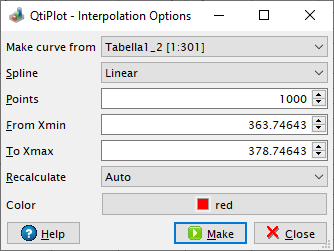# Interpolation

The interpolation command will create a new data curve with a high number of points generated by interpolation of your data. The dialog box allows you to define the number of output data points (default value is 1000), the method used for interpolation, the interval of X-values to interpolate over and the color of the interpolated curve. In addition to the new curve in the active plot, a new table will be created containing the data for the curve.Three interpolation methods are available. The first is a linear method. In this case, interpolated data points are placed along the straight between every two adjacent values of your data. The second is the method of natural cubic splines. The word "natural" meaning that the second derivatives of the spline polynomials are set equal to zero at the endpoints of the interval of interpolation. The last method, Akima, is a polynomial interpolation that requires a minimum of five data points in the curve to be interpolated.

Please note that using these interpolation methods it is also posible to extrapolate values bellow Xmin (linear and cubic methods only) and beyond Xmax for all three methods.

Figure 6-18. Comparison of the three methods of interpolation